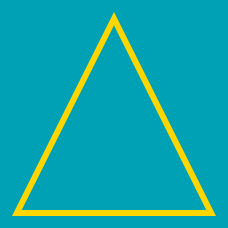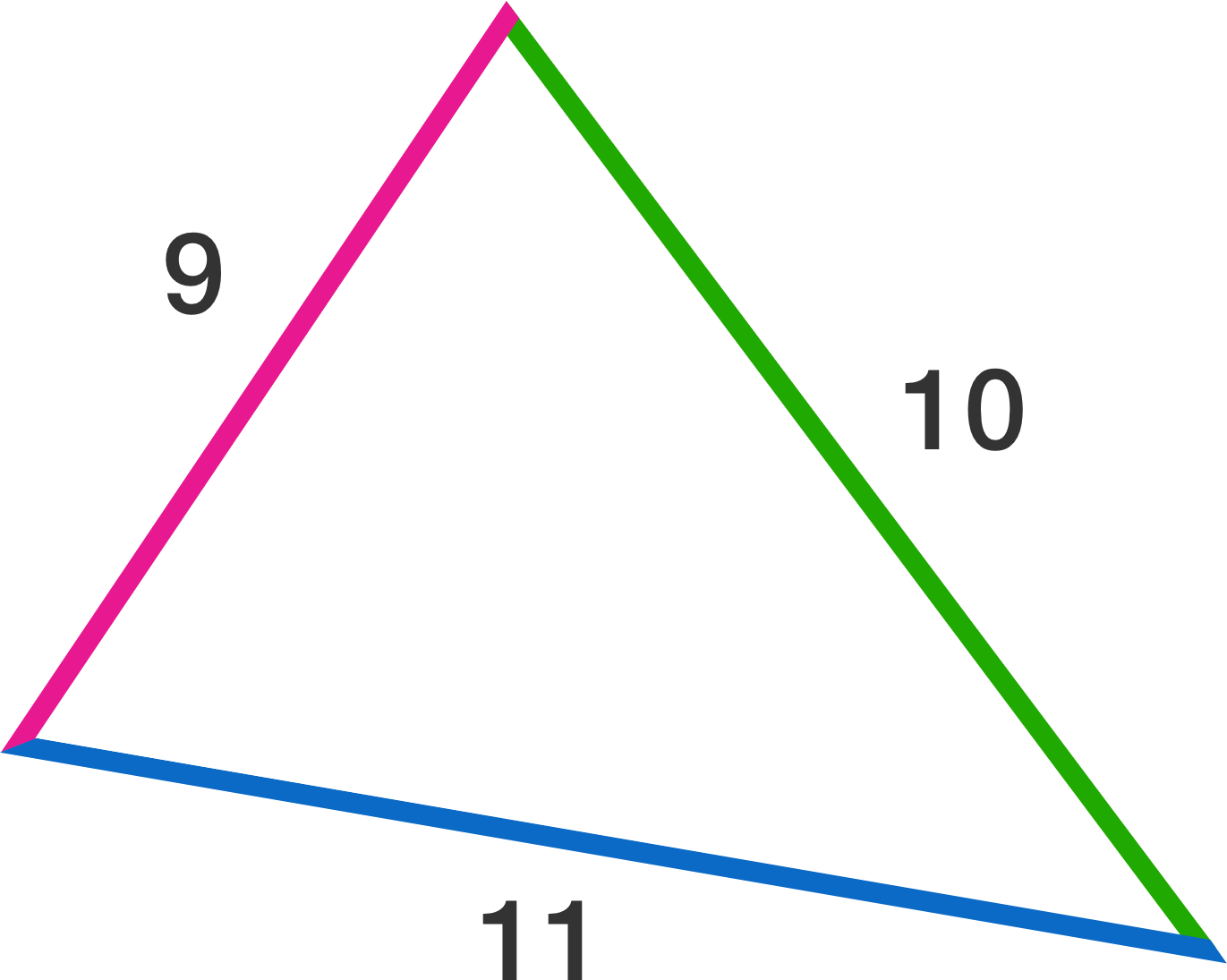Geometry

# Area of Triangles - Heron's Formula

What is the area of a triangle with side lengths $9,12,$ and $15?$

What is the area of a triangle with side lengths $13, 14,$ and $15?$

What is the area of a triangle with side lengths $9, 11,$ and $10?$A triangle has perimeter 14 and area $2\sqrt{14}.$ If the shortest side has length 3, find the positive difference between the lengths of other two sides. Give your answer to 3 decimal places.

What is the area of a triangle with side lengths $3,5,$ and $7?$

×